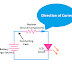The direction of the current is opposite to the electrons. That means the electrical current flows in the opposite direction as the electrons flow. From the convention, it can be said that electric current flows from the higher potential to the lower potential while electrons flow from the lower potential to the higher potential. In the case of DC, there is constant voltage and polarity also does not change. So, here electric current flows from positive to negative. In actuality, nobody has seen the flow of current visually and it is not possible also All the theories are based on the convention researched by the scientists.

In the case of AC or Alternating Current, the magnitude and direction change with time. So, the direction of the current also changes. When an AC circuit consists of passive elements such as inductors, capacitor then the waveform of the voltage and current changes. When the AC circuit consists of a pure capacitor, then the current leads behind the voltage while if the AC circuit consists of inductors the current lags behind the voltage.

Now come to the topic that is how to control the flow and direction of the current. It can be understood easily if we know the working principle of a rectifier circuit. Basically, the rectifier is an electrical or electronic circuit that converts the alternating current into direct current. In AC, the current changes its direction continuously but in DC current flows in a constant direction. The rectifier circuit makes the flow of current in one direction only.

Remember that current only flows when the circuit is in closed condition. When a potential difference or voltage is applied to a closed circuit, the current starts to flow from the higher potential to the lower potential. Current never flows, if there is any broken or open in a circuit. Also, the flow of current is opposed by a resistor or internal resistance of the conductive wire or path.

What is the direction of Current? ExplainedReviewed by Author on January 19, 2022 Rating: 5